# The consistent tangent matrix

Home » Knowledge Base » General » Materials » The consistent tangent matrix

In the evaluation of the tangent sti ness matrix, the material tangent matrix is required. The continuum tangent matrix was developed in a previous article, and it is frequently used in nite element calculations.

A slightly di erent tangent matrix, however, is derived by di erentiating the radial return stress update with respect to the increment in the strain. The nite element literature refers to the latter matrix as the consistent tangent matrix because it is consistent with the radial return algorithm. The di erence between the two tangent matrices only appears in the terms associated with the deviatoric stress since the mean stress is updated elastically with radial return. Calculations performed with full Newton iteration exhibit quadratic convergence more frequently with the consistent tangent than the continuum tangent. However, nite element iterations only converge quadratically near the solution. When large load increments are applied, most of the iterations will converge at a linear rate at best regardless of the tangent. The bene t with a quasi-Newton method is less clear. For clarity and to make its implementation more straight forward, the derivation is presented using index notation, and σ (σ^Tr) is simpli ed to σ . Since the mean stress term is elastic, and, therefore its tangent is simply the associated elastic terms, only the deviatoric stress is considered.The tangent matrix is evaluated by using the chain rule of di erentiation,Evaluating the rst derivative on the right hand side,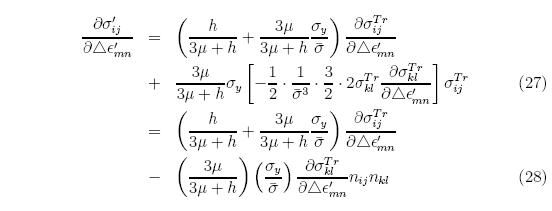where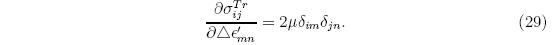Substituting Equation 29 into Equation 28 gives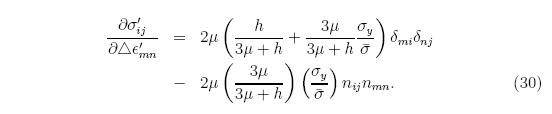The deviatoric strain increment is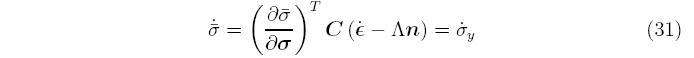and, therefore, the second term in Equation 26 isAdding the term for the mean strain, substituting Equation 32 into Equation 30, and simplifying, gives the nal result,This tangent is only used when there is plastic the elastic tangent is used. Note that as the strain increment approaches zero, the ratio σ y= σ approaches unity, and therefore the rst two terms on the right hand side become the standard tangent for linear, isotropic elasticity, and the consistent tangent becomes equal to the continuum tangent. Some numerical di culties, associated with the ill-conditioning of the consistent tangent, can occur when simultaneously the hardening, h, approaches zero, and the strain increment becomes large, making the ratio σ y= σ small. Letting h go to zero, the tangent becomesand letting σ y= σ approach zero then gives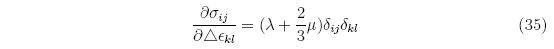which is simply the term associated with the pressure, i.e., all the terms associated with plasticity have gone to zero.

djb 2005